# Concept of lists and array implementations of lists

• Slides: 61
Download presentationConcept of lists and array implementations of listsA simple ‘list’ example Concept of a list, e. g. a list of integers * A list is a linear sequence of objects * * n n Print out info Empty test n n n Search Insertion Deletion n … implemented by a static array (over-sized if necessary) int list; int size; n by a dynamic array int list[size]; int size; n by a linked list and more … n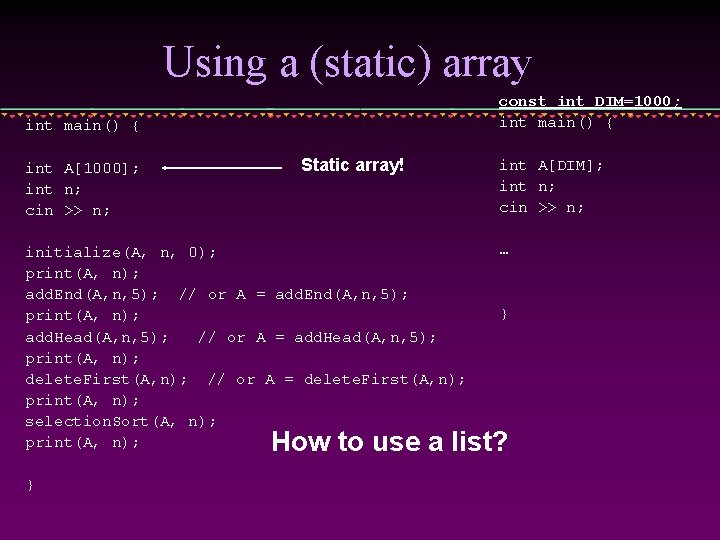Using a (static) array const int DIM=1000; int main() { int A; int n; cin >> n; Static array! initialize(A, n, 0); print(A, n); add. End(A, n, 5); // or A = add. End(A, n, 5); print(A, n); add. Head(A, n, 5); // or A = add. Head(A, n, 5); print(A, n); delete. First(A, n); // or A = delete. First(A, n); print(A, n); selection. Sort(A, n); print(A, n); int A[DIM]; int n; cin >> n; … } How to use a list? }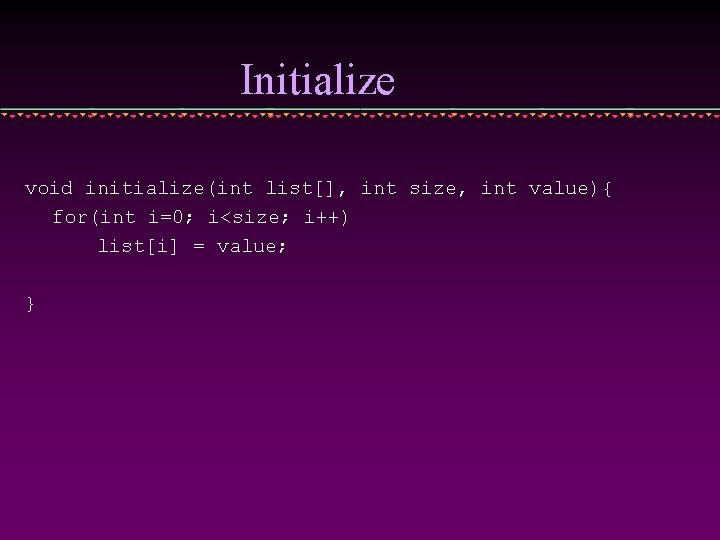Initialize void initialize(int list[], int size, int value){ for(int i=0; i<size; i++) list[i] = value; }Print out a list void print(int list[], int size) { cout << "[ "; for(int i=0; i<size; i++) cout << list[i] << " "; cout << "]" << endl; }Delete the first element // for deleting the first element of the array void delete. First(int list[], int& size){ for(int i=0; i<size-1; i++) list[i] = list[i+1]; delete. First(A, n) size--; } int* delete. First(int list[], int& size){ Pointer type! for(int i=0; i<size-1; i++) list[i] = list[i+1]; size--; return list; } B = delete. First(A, n)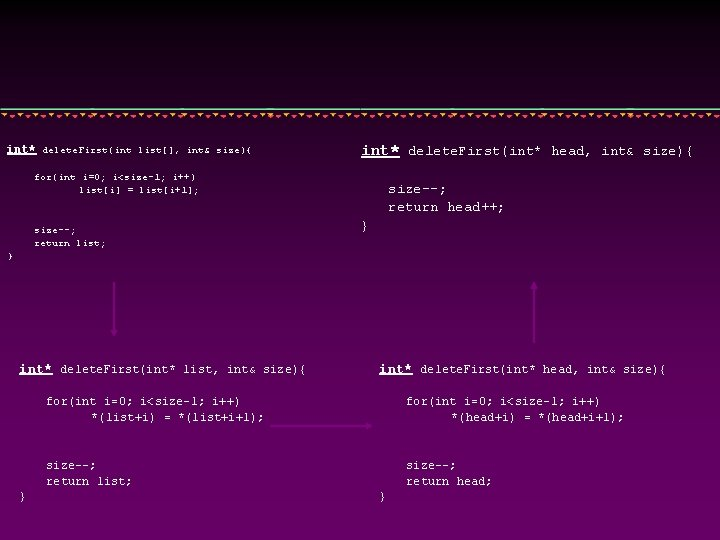int* delete. First(int list[], int& size){ int* delete. First(int* head, int& size){ for(int i=0; i<size-1; i++) list[i] = list[i+1]; size--; return list; size--; return head++; } } int* delete. First(int* list, int& size){ } int* delete. First(int* head, int& size){ for(int i=0; i<size-1; i++) *(list+i) = *(list+i+1); for(int i=0; i<size-1; i++) *(head+i) = *(head+i+1); size--; return list; size--; return head; }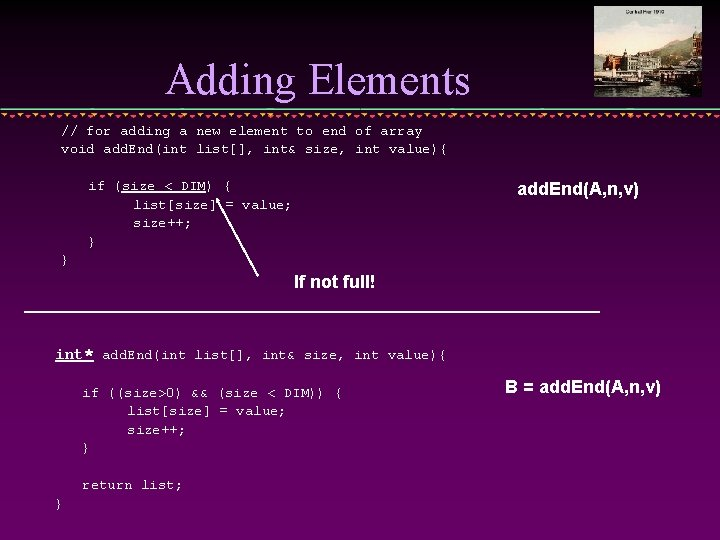Adding Elements // for adding a new element to end of array void add. End(int list[], int& size, int value){ if (size < DIM) { list[size] = value; size++; } add. End(A, n, v) } If not full! int* add. End(int list[], int& size, int value){ if ((size>0) && (size < DIM)) { list[size] = value; size++; } return list; } B = add. End(A, n, v)Add at the beginning: // for adding a new element at the beginning of the array void add. Head(int list[], int& size, int value){ if(size < DIM){ for(int i=size-1; i>0; i--) list[i+1] = list[i]; list = value; size++; add. Head(A, n, v) } else cout << “out of array memory!!! << endl; } int* add. Head(int list[], int& size, int value){ if(size < DIM){ for(int i=size-1; i>0; i--) list[i+1] = list[i]; list = value; size++; return list; } else … } B = add. Head(A, n, v)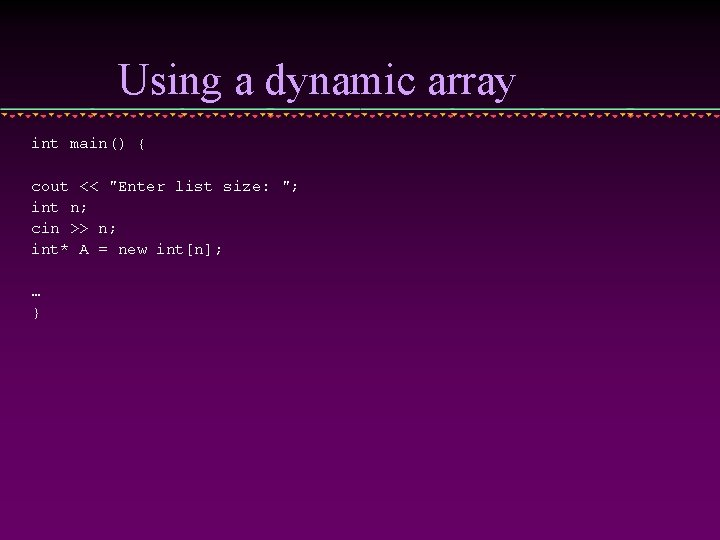Using a dynamic array int main() { cout << "Enter list size: "; int n; cin >> n; int* A = new int[n]; … }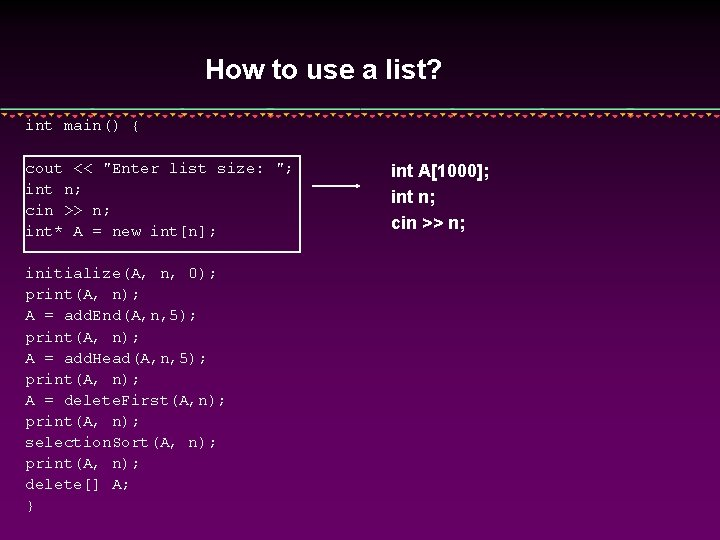How to use a list? int main() { cout << "Enter list size: "; int n; cin >> n; int* A = new int[n]; initialize(A, n, 0); print(A, n); A = add. End(A, n, 5); print(A, n); A = add. Head(A, n, 5); print(A, n); A = delete. First(A, n); print(A, n); selection. Sort(A, n); print(A, n); delete[] A; } int A; int n; cin >> n;Initialize and Print-out: the same as before void initialize(int list[], int size, int value){ for(int i=0; i<size; i++) list[i] = value; } void print(int list[], int size) { cout << "[ "; for(int i=0; i<size; i++) cout << list[i] << " "; cout << "]" << endl; }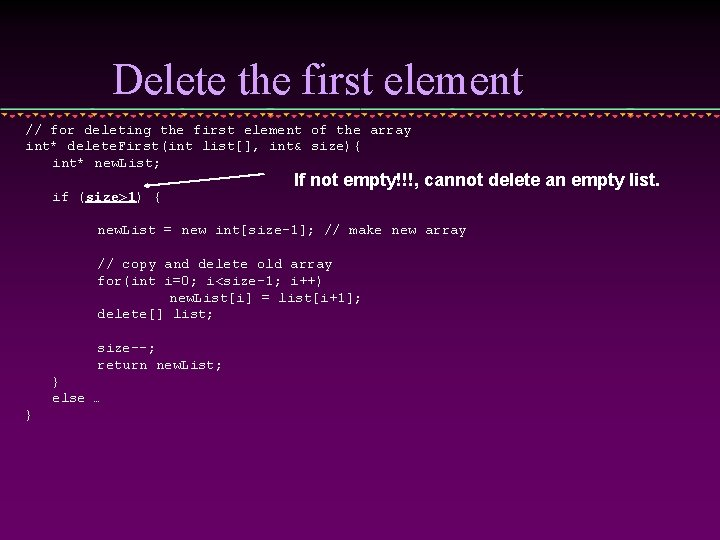Delete the first element // for deleting the first element of the array int* delete. First(int list[], int& size){ int* new. List; if (size>1) { If not empty!!!, cannot delete an empty list. new. List = new int[size-1]; // make new array // copy and delete old array for(int i=0; i<size-1; i++) new. List[i] = list[i+1]; delete[] list; size--; return new. List; } else … }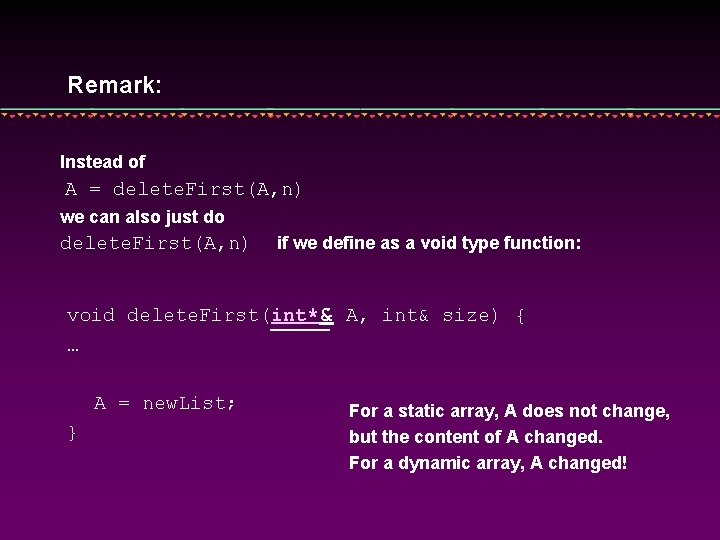Remark: Instead of A = delete. First(A, n) we can also just do delete. First(A, n) if we define as a void type function: void delete. First(int*& A, int& size) { … A = new. List; } For a static array, A does not change, but the content of A changed. For a dynamic array, A changed!Adding Elements // for adding a new element to end of array int* add. End(int list[], int& size, int value){ int* new. List; new. List = new int [size+1]; // make new array if(size){ // copy and delete old array for(int i=0; i<size; i++) new. List[i] = list[i]; delete[] list; } Not empty list new. List[size] = value; size++; return new. List; }Add at the beginning: // for adding a new element at the beginning of the array int* add. Head(int list[], int& size, int value){ int* new. List; new. List = new int [size+1]; // make new array if(size){ // copy and delete old array for(int i=0; i<size; i++) new. List[i+1] = list[i]; delete[] list; } new. List = value; size++; return new. List; }Search and delete … // for adding a new element to end of array int* delete(int list[], int& size, int value){ Search the element in the array and get the position Shift all elements after it towards this position … }Linked ListsMotivation * A “List” is a useful structure to hold a collection of data. n * Currently, we use arrays for lists Examples: List of ten students marks int student. Marks; List of temperatures for the last two weeks double temperature;Motivation * list using static array int my. Array; int n; We have to decide (to oversize) in advance the size of the array (list) * list using dynamic array int* my. Array; int n; cin >> n; my. Array = new int[n]; We allocate an array (list) of any specified size while the program is running * linked-list (dynamic size) size = ? ? The list is dynamic. It can grow and shrink to any size.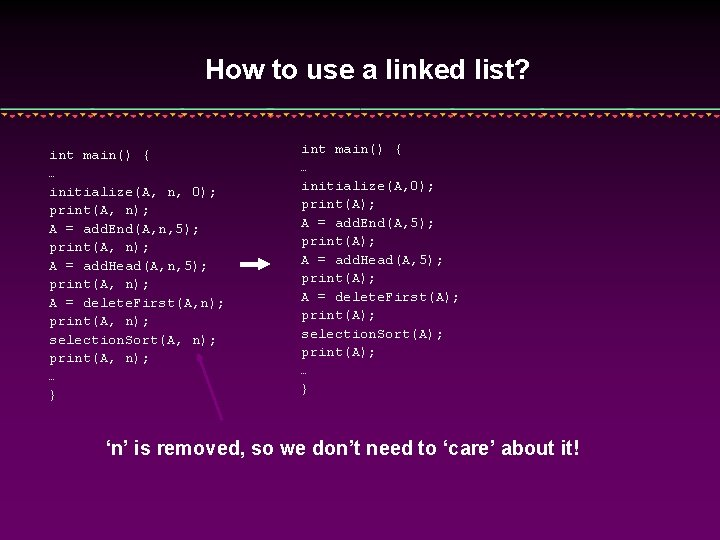How to use a linked list? int main() { … initialize(A, n, 0); print(A, n); A = add. End(A, n, 5); print(A, n); A = add. Head(A, n, 5); print(A, n); A = delete. First(A, n); print(A, n); selection. Sort(A, n); print(A, n); … } int main() { … initialize(A, 0); print(A); A = add. End(A, 5); print(A); A = add. Head(A, 5); print(A); A = delete. First(A); print(A); selection. Sort(A); print(A); … } ‘n’ is removed, so we don’t need to ‘care’ about it!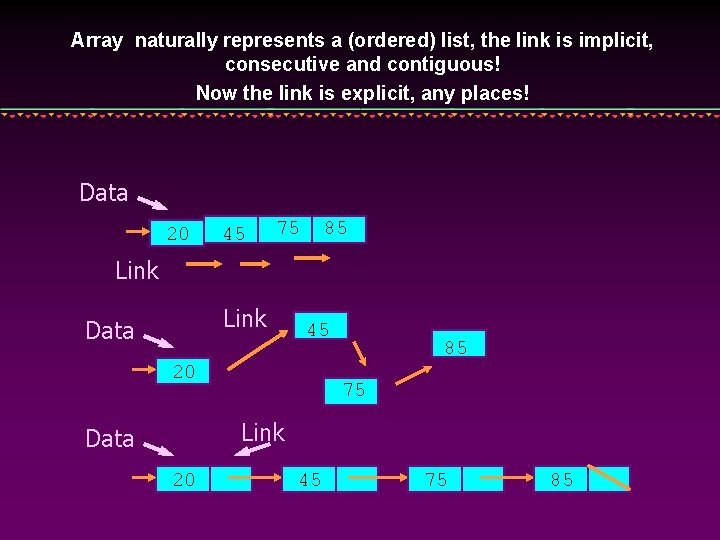Array naturally represents a (ordered) list, the link is implicit, consecutive and contiguous! Now the link is explicit, any places! Data 20 45 75 85 Link Data 45 20 85 75 Link Data 20 45 75 85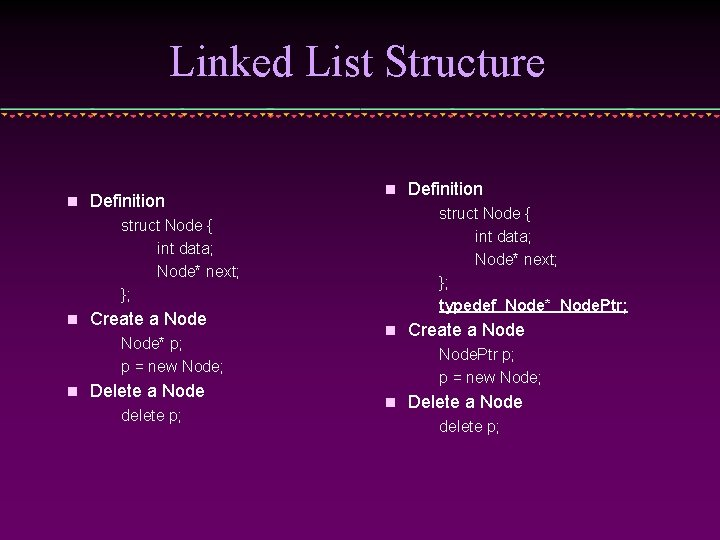Linked List Structure n Definition n struct Node { int data; Node* next; }; typedef Node* Node. Ptr; struct Node { int data; Node* next; }; n Create a Node* p; p = new Node; n Delete a Node delete p; Definition n Create a Node. Ptr p; p = new Node; n Delete a Node delete p;n Access fields in a node (*p). data; //access the data field (*p). next; //access the pointer field Or it can be accessed this way p->data //access the data field p->next //access the pointer fieldRepresenting and accessing linked lists Head 20 * 45 75 85 We define a pointer Node. Ptr head; (also: Node* head) that points to the first node of the linked list. When the linked list is empty then head is NULL.Passing a Linked List to a Function It is roughly the same as for an array!!! * When passing a linked list to a function it should suffice to pass the value of head. Using the value of head the function can access the entire list. * Problem: If a function changes the beginning of a list by inserting or deleting a node, then head will no longer point to the beginning of the list. * Solution: When passing head always pass it by reference or using a function to return a new pointer value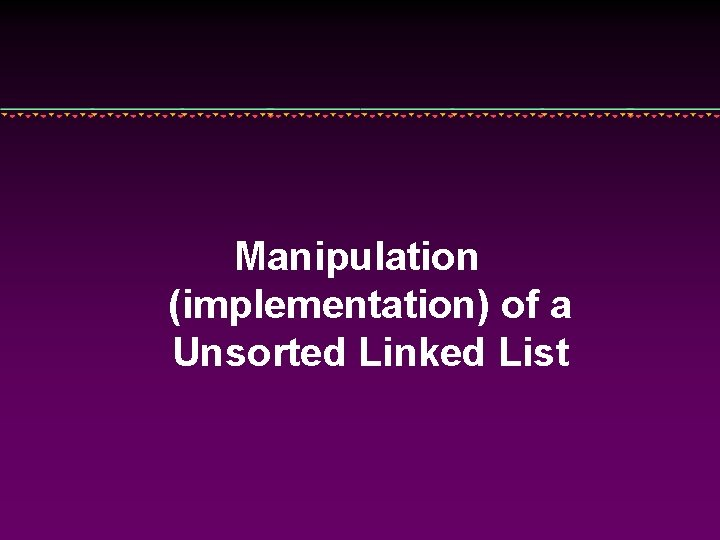Manipulation (implementation) of a Unsorted Linked ListStart the first node from scratch head = NULL; Head Node. Ptr new. Ptr; new. Ptr = new Node; new. Ptr->data = 20; new. Ptr->next = NULL; head = new. Ptr; 20 Head new. PtrInserting a Node at the Beginning new. Ptr = new Node; new. Ptr->data = 13; new. Ptr->next = Head; head = new. Ptr; 20 Head 13 new. PtrKeep going … Head 50 new. Ptr 40 13 20Adding an element to the head: void add. Head(Node. Ptr& head, int newdata){ Node. Ptr new. Ptr = new Node; new. Ptr->data = newdata; new. Ptr->next = Head; head = new. Ptr; }It can also be written (more functionally) as: Node. Ptr add. Head(Node. Ptr head, int newdata){ Node. Ptr new. Ptr = new Node; new. Ptr->data = newdata; new. Ptr->next = Head; return new. Ptr; } Compare it with ‘add. Head’ with a dynamic array implementationDeleting the Head Node. Ptr p; p = head; head = head->next; delete p; head (to delete) 50 p 40 13 20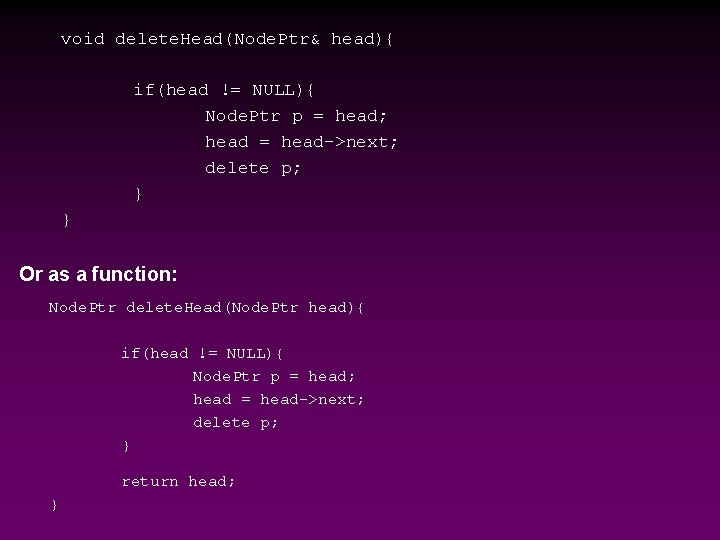void delete. Head(Node. Ptr& head){ if(head != NULL){ Node. Ptr p = head; head = head->next; delete p; } } Or as a function: Node. Ptr delete. Head(Node. Ptr head){ if(head != NULL){ Node. Ptr p = head; head = head->next; delete p; } return head; }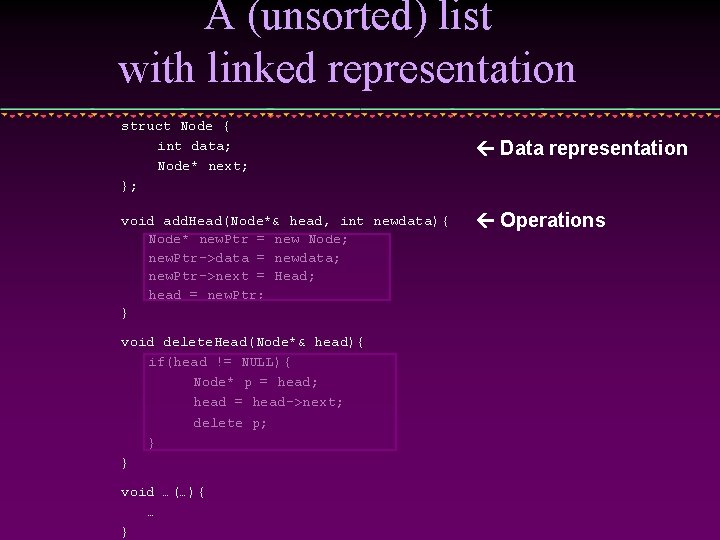A (unsorted) list with linked representation struct Node { int data; Node* next; }; void add. Head(Node*& head, int newdata){ Node* new. Ptr = new Node; new. Ptr->data = newdata; new. Ptr->next = Head; head = new. Ptr; } void delete. Head(Node*& head){ if(head != NULL){ Node* p = head; head = head->next; delete p; } } void …(…){ … } Data representation Operations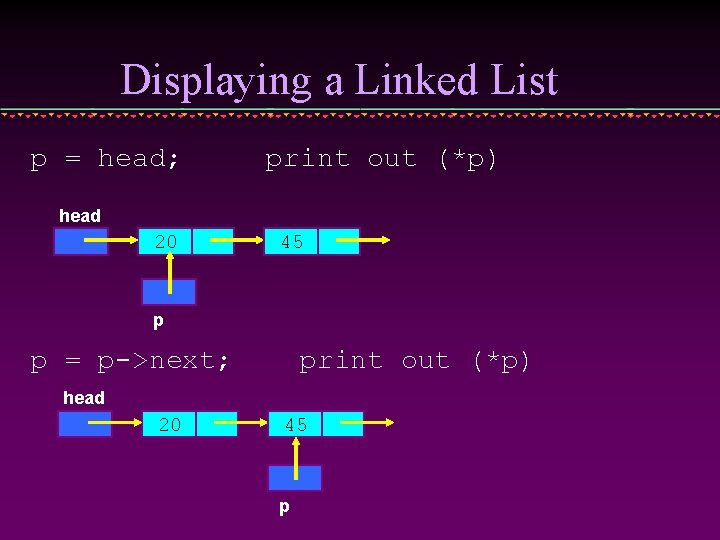Displaying a Linked List p = head; print out (*p) head 20 45 p p = p->next; print out (*p) head 20 45 p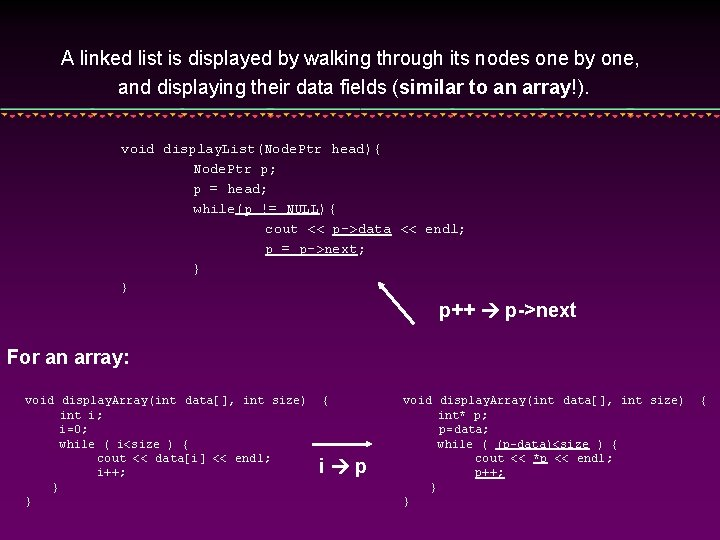A linked list is displayed by walking through its nodes one by one, and displaying their data fields (similar to an array!). void display. List(Node. Ptr head){ Node. Ptr p; p = head; while(p != NULL){ cout << p->data << endl; p = p->next; } } p++ p->next For an array: void display. Array(int data[], int size) int i; i=0; while ( i<size ) { cout << data[i] << endl; i++; } } { i p void display. Array(int data[], int size) int* p; p=data; while ( (p-data)<size ) { cout << *p << endl; p++; } } {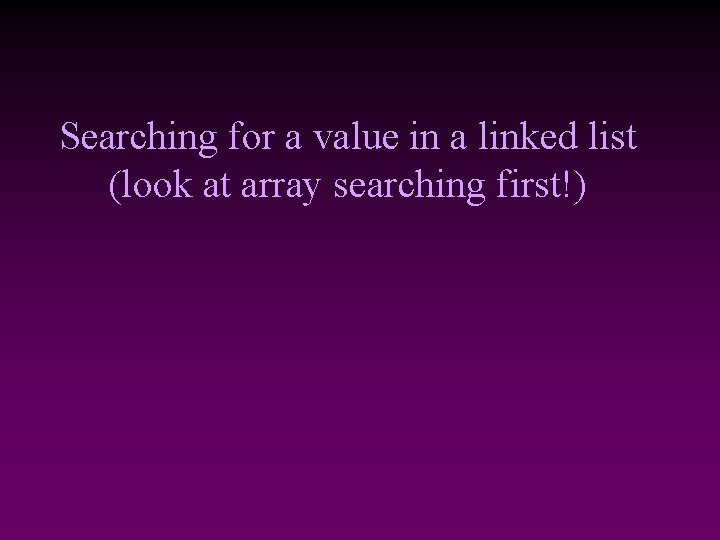Searching for a value in a linked list (look at array searching first!)Remember searching algorithm in an array: void main() { const int size=8; int data[size] = { 10, 7, 9, 1, 17, 30, 5, 6 }; int value; cout << "Enter search element: "; cin >> value; int n=0; int position=-1; bool found=false; while ( (n<size) && (!found) ) { if(data[n] == value) { found=true; position=n; } n++; } if(position==-1) cout << "Not found!!n"; else cout << "Found at: " << position << endl; } It is essentially the same!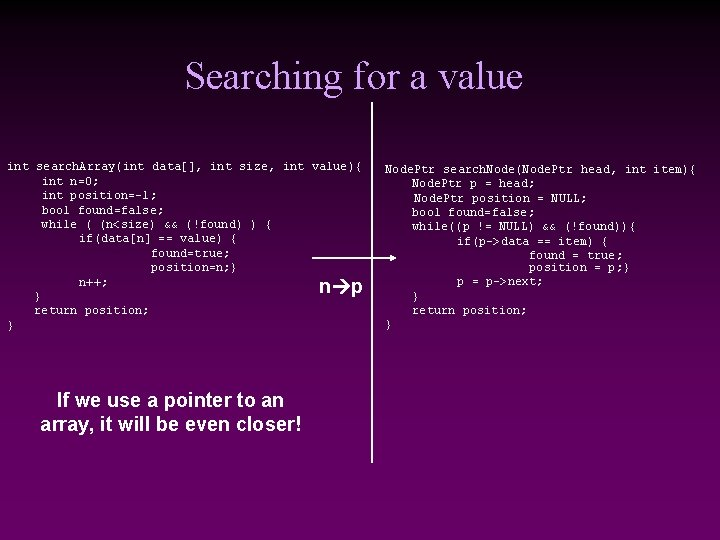Searching for a value int search. Array(int data[], int size, int value){ int n=0; int position=-1; bool found=false; while ( (n<size) && (!found) ) { if(data[n] == value) { found=true; position=n; } n++; } return position; } n p If we use a pointer to an array, it will be even closer! Node. Ptr search. Node(Node. Ptr head, int item){ Node. Ptr p = head; Node. Ptr position = NULL; bool found=false; while((p != NULL) && (!found)){ if(p->data == item) { found = true; position = p; } p = p->next; } return position; }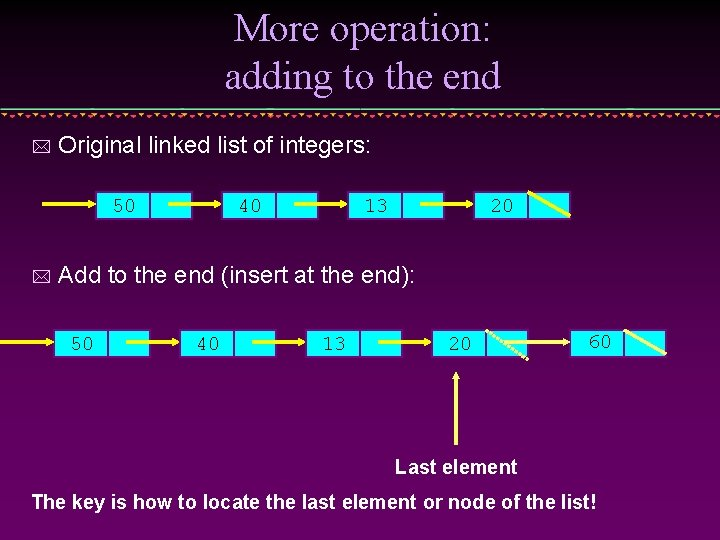More operation: adding to the end * Original linked list of integers: 50 * 40 13 20 Add to the end (insert at the end): 50 40 13 20 60 Last element The key is how to locate the last element or node of the list!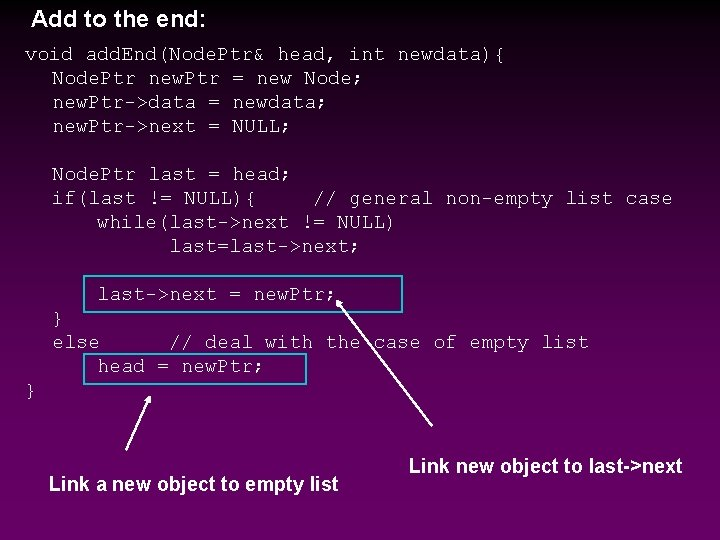Add to the end: void add. End(Node. Ptr& head, int newdata){ Node. Ptr new. Ptr = new Node; new. Ptr->data = newdata; new. Ptr->next = NULL; Node. Ptr last = head; if(last != NULL){ // general non-empty list case while(last->next != NULL) last=last->next; last->next = new. Ptr; } else // deal with the case of empty list head = new. Ptr; } Link a new object to empty list Link new object to last->next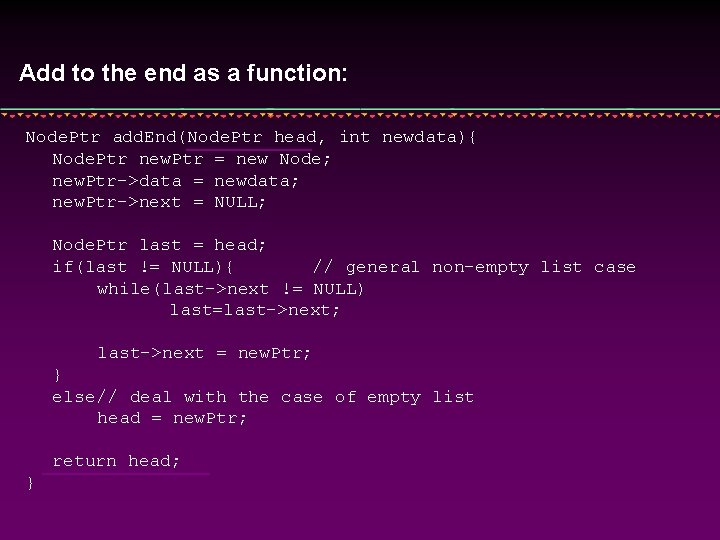Add to the end as a function: Node. Ptr add. End(Node. Ptr head, int newdata){ Node. Ptr new. Ptr = new Node; new. Ptr->data = newdata; new. Ptr->next = NULL; Node. Ptr last = head; if(last != NULL){ // general non-empty list case while(last->next != NULL) last=last->next; last->next = new. Ptr; } else // deal with the case of empty list head = new. Ptr; return head; }Manipulation (implementation) of a Sorted Linked List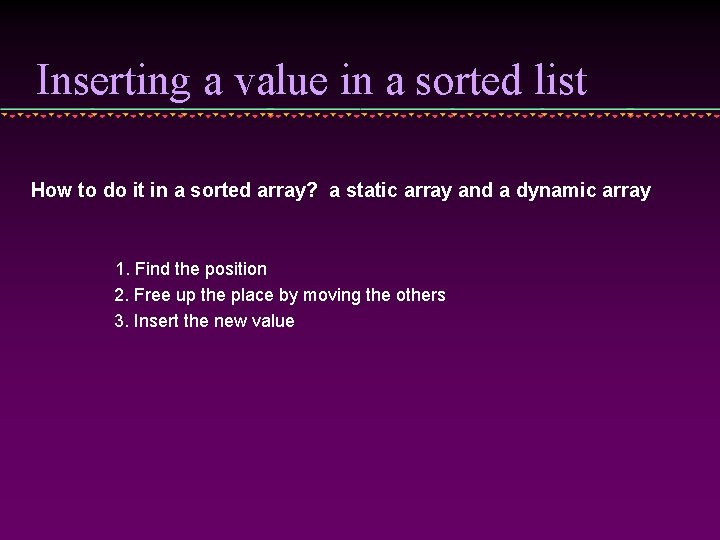Inserting a value in a sorted list How to do it in a sorted array? a static array and a dynamic array 1. Find the position 2. Free up the place by moving the others 3. Insert the new value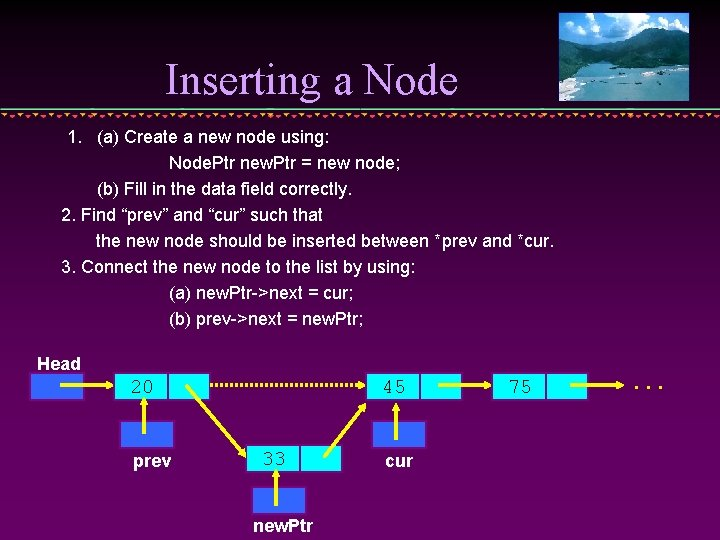Inserting a Node 1. (a) Create a new node using: Node. Ptr new. Ptr = new node; (b) Fill in the data field correctly. 2. Find “prev” and “cur” such that the new node should be inserted between *prev and *cur. 3. Connect the new node to the list by using: (a) new. Ptr->next = cur; (b) prev->next = new. Ptr; Head 20 prev 45 33 new. Ptr cur 75 . . .Finding prev and cur Suppose that we want to insert or delete a node with data value new. Value. Then the following code successfully finds prev and cur such that prev->data < new. Value <= cur->data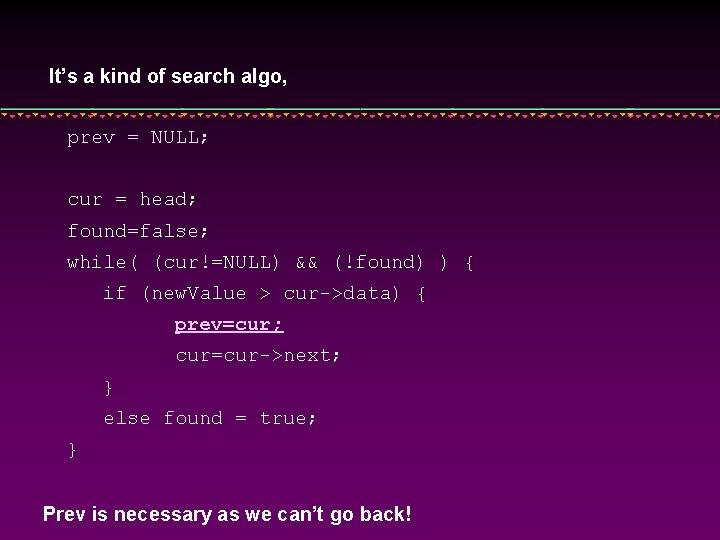It’s a kind of search algo, prev = NULL; cur = head; found=false; while( (cur!=NULL) && (!found) ) { if (new. Value > cur->data) { prev=cur; cur=cur->next; } else found = true; } Prev is necessary as we can’t go back!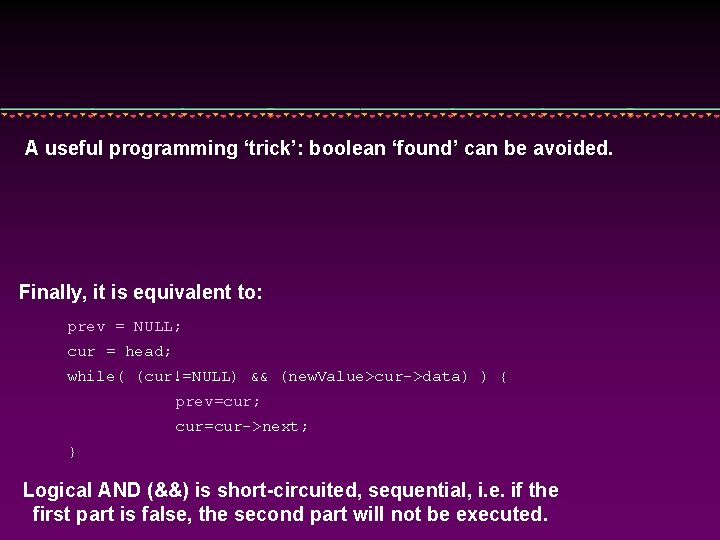A useful programming ‘trick’: boolean ‘found’ can be avoided. Finally, it is equivalent to: prev = NULL; cur = head; while( (cur!=NULL) && (new. Value>cur->data) ) { prev=cur; cur=cur->next; } Logical AND (&&) is short-circuited, sequential, i. e. if the first part is false, the second part will not be executed.//insert item into linked list according to ascending order void insert. Node(Node. Ptr& head, int item){ Node. Ptr newp, cur, pre; newp = new Node; newp->data = item; pre = NULL; cur = head; while( (cur != NULL) && (item>cur->data)){ pre = cur; cur = cur->next; } if(pre == NULL){ //insert to head of linked list newp->next = head; If the position happens to be the head = newp; } else { pre->next = newp; new->next = cur; General case } }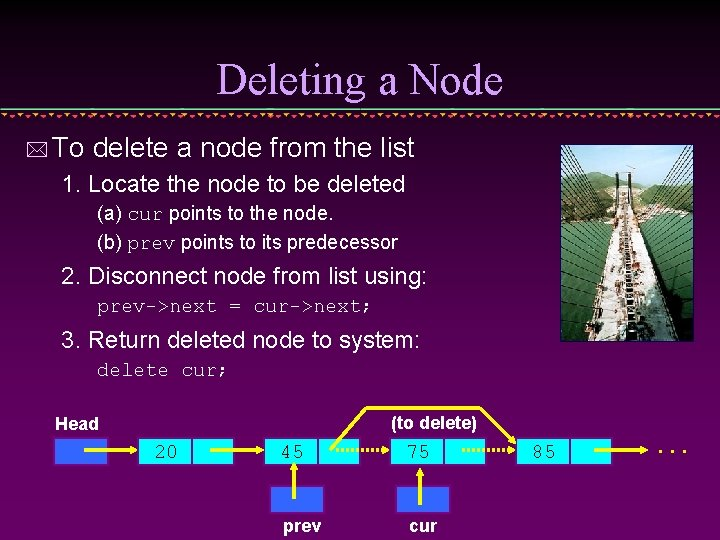Deleting a Node * To delete a node from the list 1. Locate the node to be deleted (a) cur points to the node. (b) prev points to its predecessor 2. Disconnect node from list using: prev->next = cur->next; 3. Return deleted node to system: delete cur; (to delete) Head 20 45 75 prev cur 85 . . .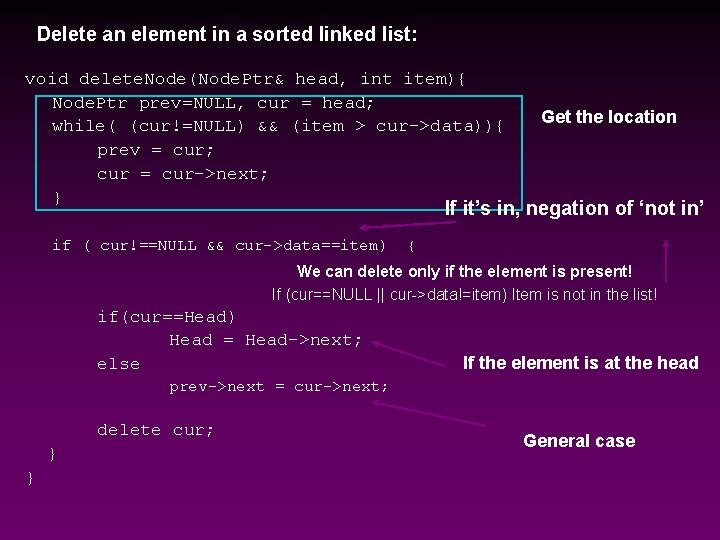Delete an element in a sorted linked list: void delete. Node(Node. Ptr& head, int item){ Node. Ptr prev=NULL, cur = head; while( (cur!=NULL) && (item > cur->data)){ prev = cur; cur = cur->next; } Get the location If it’s in, negation of ‘not in’ if ( cur!==NULL && cur->data==item) { We can delete only if the element is present! If (cur==NULL || cur->data!=item) Item is not in the list! if(cur==Head) Head = Head->next; else If the element is at the head prev->next = cur->next; delete cur; } } General caseOther variants of linked lists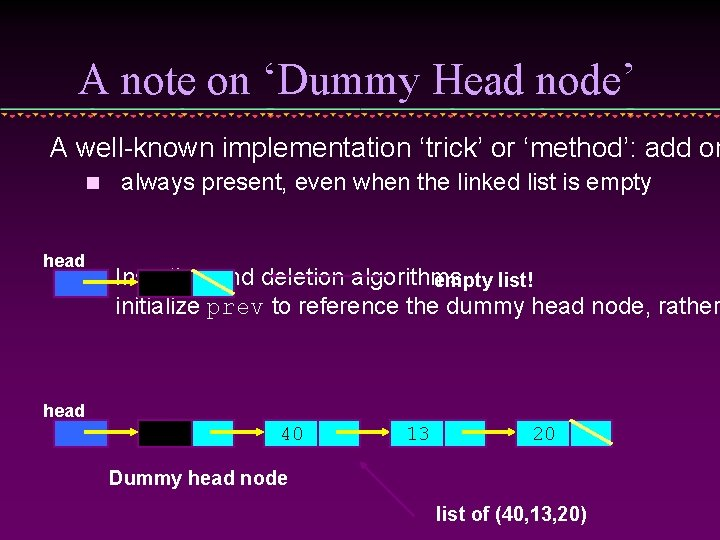A note on ‘Dummy Head node’ A well-known implementation ‘trick’ or ‘method’: add on n head n always present, even when the linked list is empty Insertion and deletion algorithms empty list! initialize prev to reference the dummy head node, rather head 40 13 20 Dummy head node list of (40, 13, 20)Circular Linked Lists A Circular Linked List is a special type of Linked List * It support the traversing from the end of the list to the beginning of the list by making the last node points back to the head of the list * 10 20 40 55 70 RearDoubly Linked Lists In a Doubly Linked-List each item points to both its predecessor and successor prev points to the predecessor n next points to the successor n 10 Head 20 Cur->prev 40 Cur 55 Cur->next 70Doubly Linked List Definition struct Node{ int data; Node* next; Node* prev; }; typedef Node* Node. Ptr;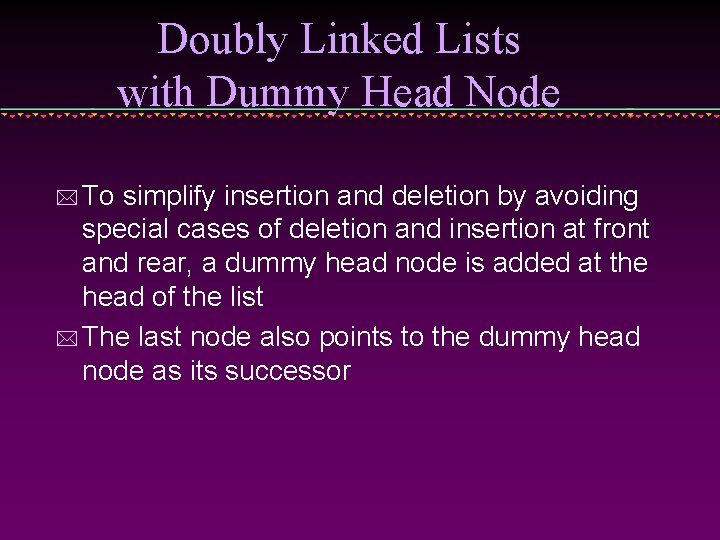Doubly Linked Lists with Dummy Head Node * To simplify insertion and deletion by avoiding special cases of deletion and insertion at front and rear, a dummy head node is added at the head of the list * The last node also points to the dummy head node as its successorIdea of ‘dummy’ object ‘dummy object’ is also called a ‘sentinel’, it allows the simplification of special cases, but confuses the emptyness NULL! l l Instead of pointing to NULL, point to the ‘dummy’!!! Skip over the dummy for the real list Dummy Head Node 10 Head 20 40 55 70Empty list: Dummy Head Node Head->next = head; compared with head=NULL;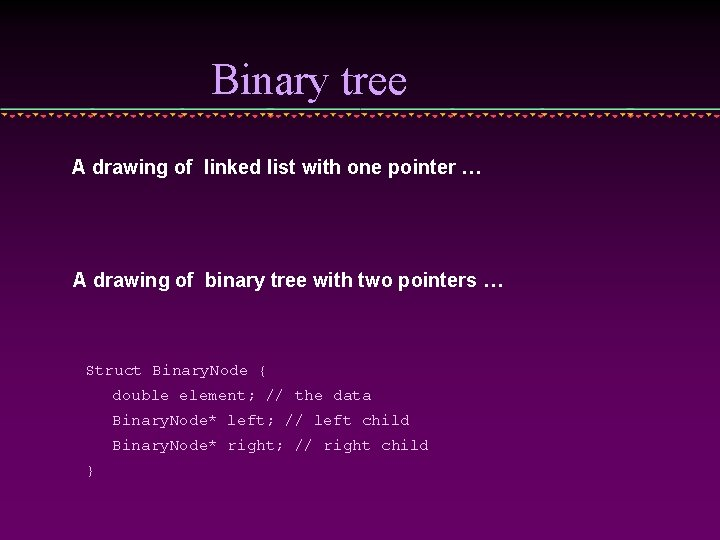Binary tree A drawing of linked list with one pointer … A drawing of binary tree with two pointers … Struct Binary. Node { double element; // the data Binary. Node* left; // left child Binary. Node* right; // right child }